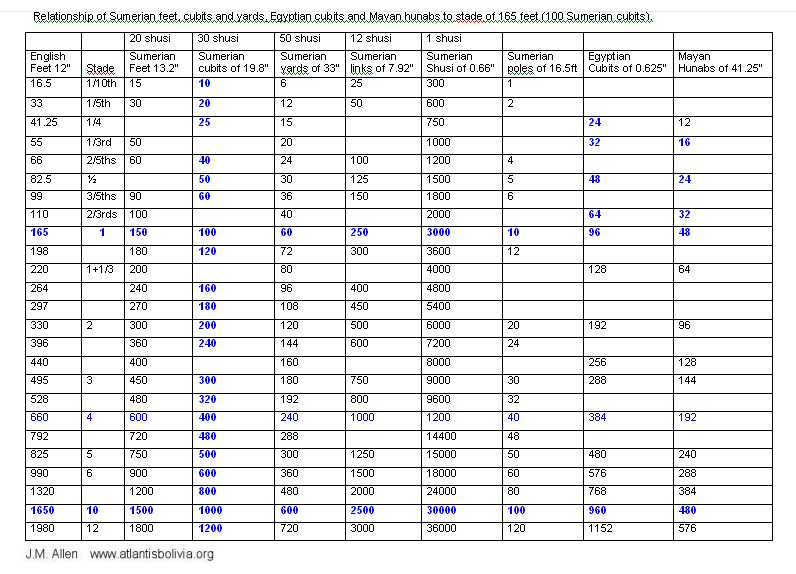### Measurement units of the Citadel in Teotihuacan, Mexico.

Satellite images provided by Google Earth have been used in this study to try and determine the units of measurement used in the planning of the enclosure known as the Citadel in the ancient city of Teotihuacan, Mexico.

This follows on from studies of measurement units in Teotihuacan and Chichen Itza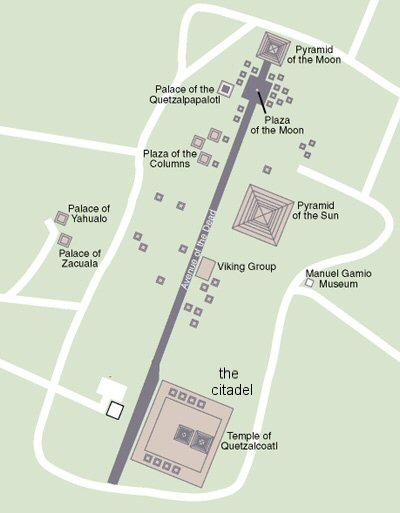Hugh Harleston jnr found Teotihuacan to be built in measures of 1.059 metres which would be 2 x cubits of 20.85" making one hunab (compare to 2 x Egyptian Royal cubits of 20.625" making 1 hunab)

Prof Sugiyama proposed a unit of 830 mm for Teotihuacan. This would be a yard of 32.68" and very interestingly compares to the "megalithic yard" of 32.64" found by Prof A. Thom for the Megalithic Sites in Britain.

Prof Sugiyama quotes a study by Drewitt (1969, 1987) and Drucker (1971,1974, 1977's) which found a unit of 805 mm (31.692").

The Sumerian basic units were a "shusi" of 0.66", link of 12 shusi (7.92"), foot of 20 shusi (13.2") cubit of 30 shusi (19.8"), yard of 50 shusi (33.0") and double yard of 100 shusi (66.0").

For larger distances, the pole of 16.5 feet (15 Sumerian feet) was used, along with a chain of 66 feet (60 Sumerian feet which was 100 links) and furlong of 660 feet (600 Sumerian feet).

Looking back to Drewitt and Druckers figure of 805 mm (31.692") we can readily see that in place of a yard of 50 shusi,they have been calculating in yards of 48 shusi (4 x links) since 48 x 0.66" = 31.68" = 804.7 mm and their study in itself provides evidence of the use of "Sumerian" measurement units at Teotihuacan.

This study looks at the citadel for evidence of "Sumerian" units, but in actual fact, it seems that "Sumerian", "Egyptian" and "Mayan" units are all based on the same measurement foundation, a plot of 100 x 100 "Sumerian" cubits which when divided by 100, 96 or 48 gives "Sumerian", Egyptian" or Mayan" units respectively.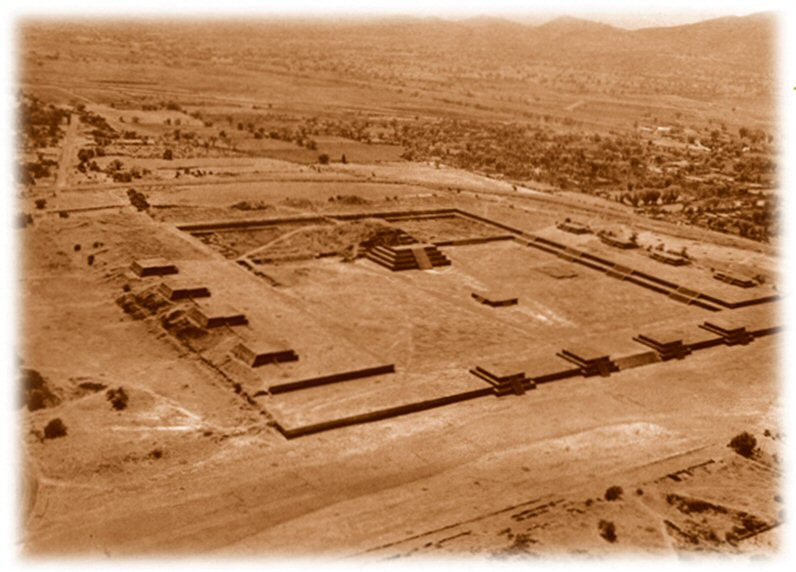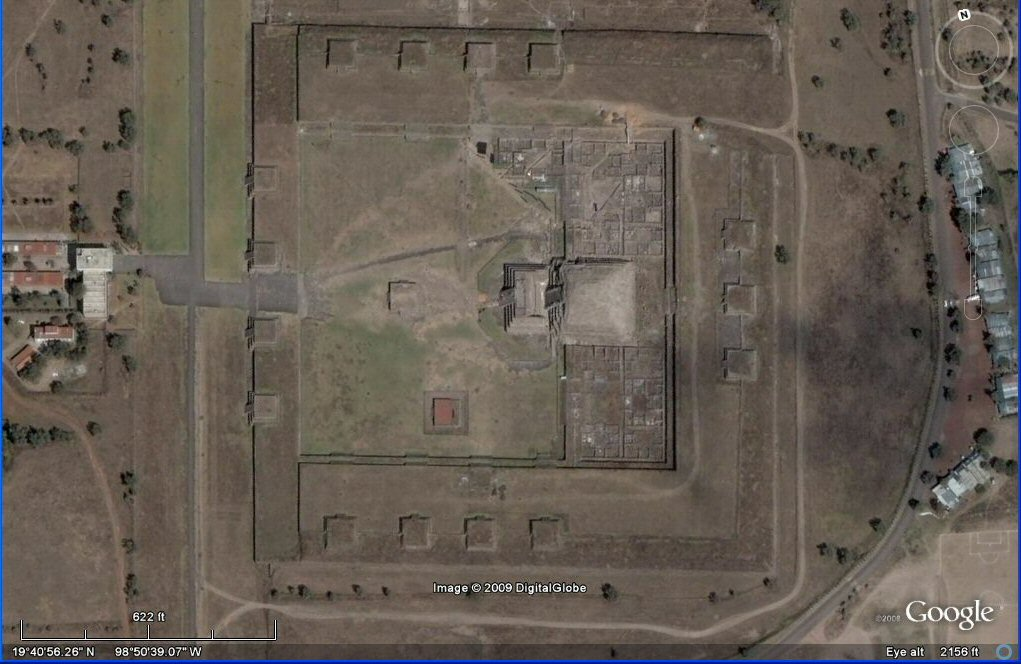Some examples of measurements at the Citadel in Sumerian feet are given below.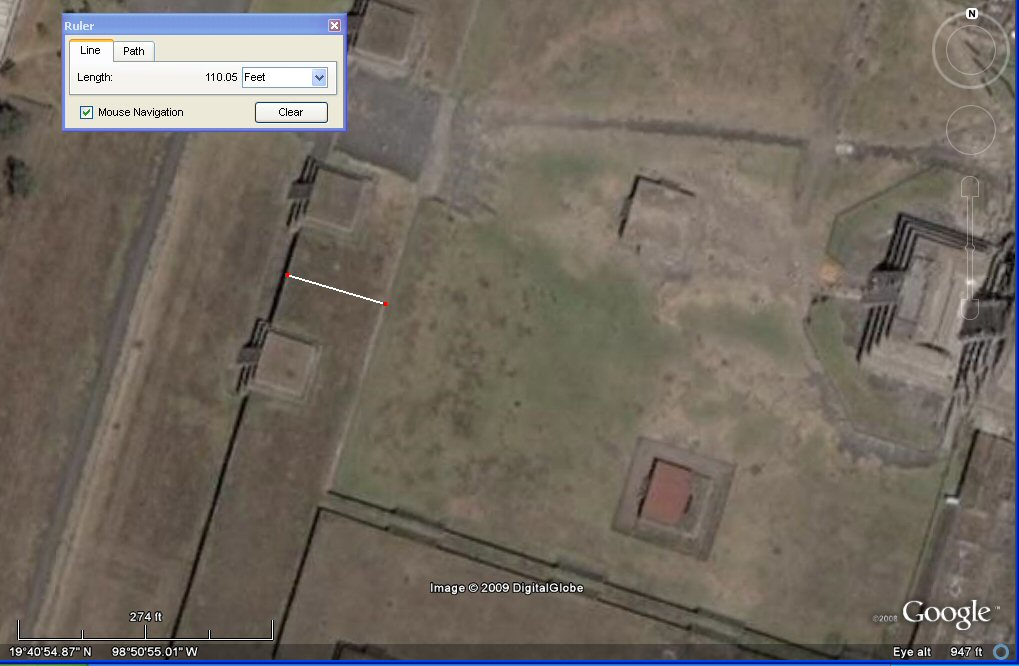above, the Google Earth satellite measures the width of the entrance wall as 110 feet -
this equals 100 Sumerian feet of 13.2", 40 Sumerian yards of 33.0", 20 Sumerian double yards of 66.0",
or 64 Egyptian royal cubits of 20.62" or 32 Mayan hunabs of 41.25" .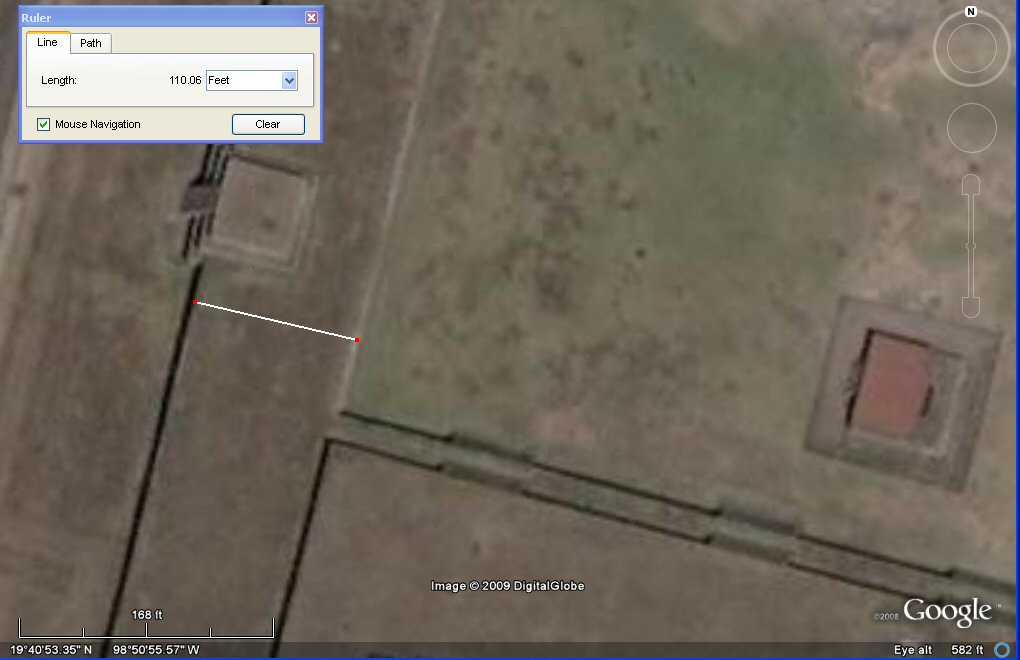above, close-up of measurement equals 100 Sumerian feet of 13.2" .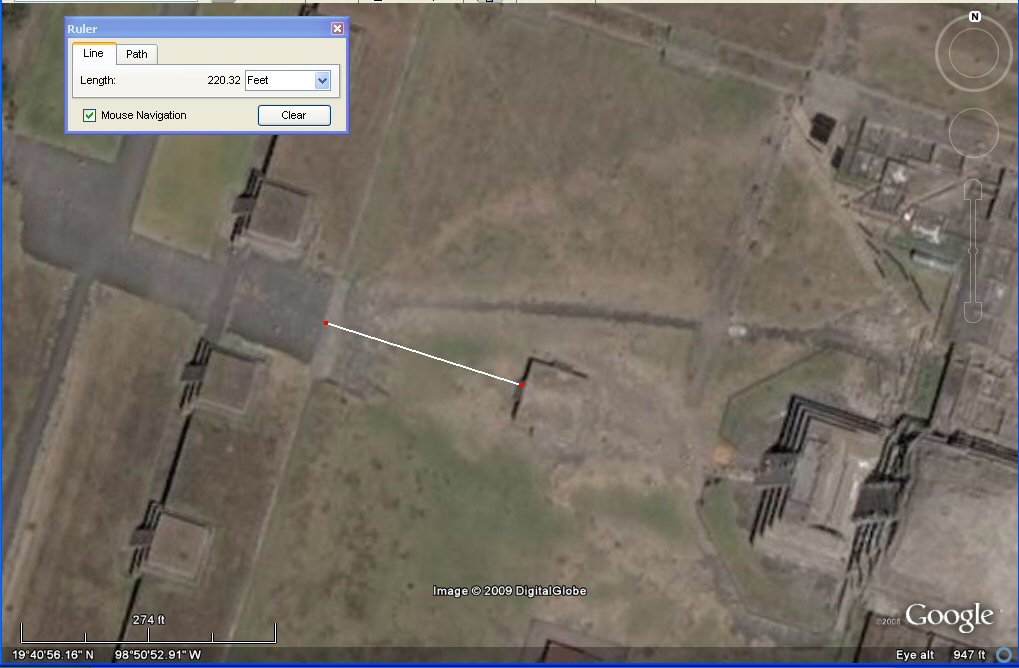above, from the entrance to the first construction is 200 Sumerian feet of 13.2"" .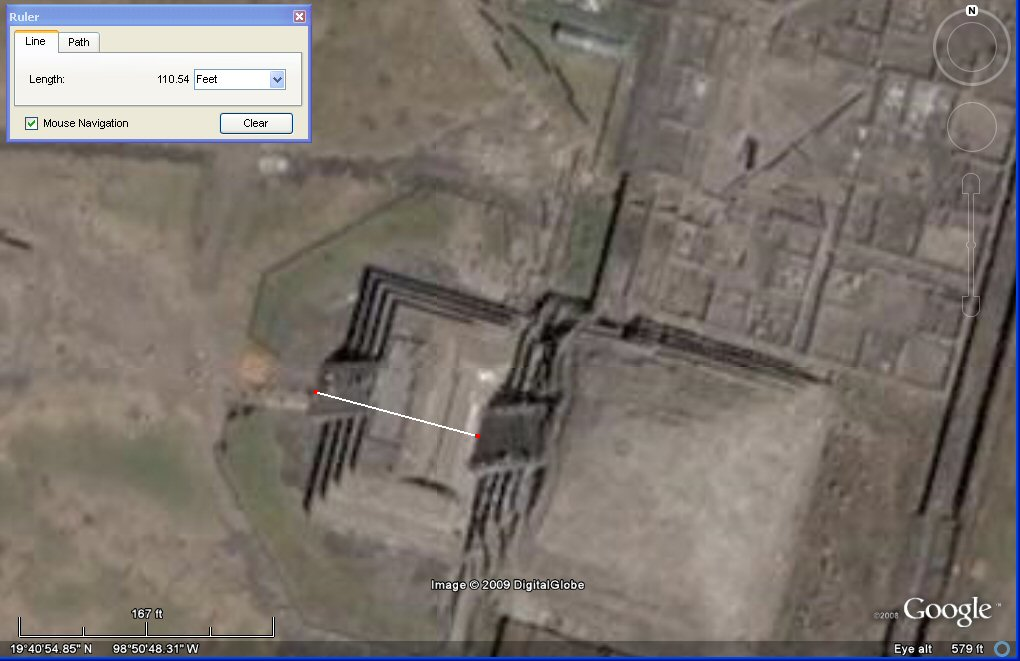above, the Google Earth satellite measures the distance from the front of the front pyramid platform steps to the front of the rear pyramid steps as 110 English feet = 100 Sumerian feet.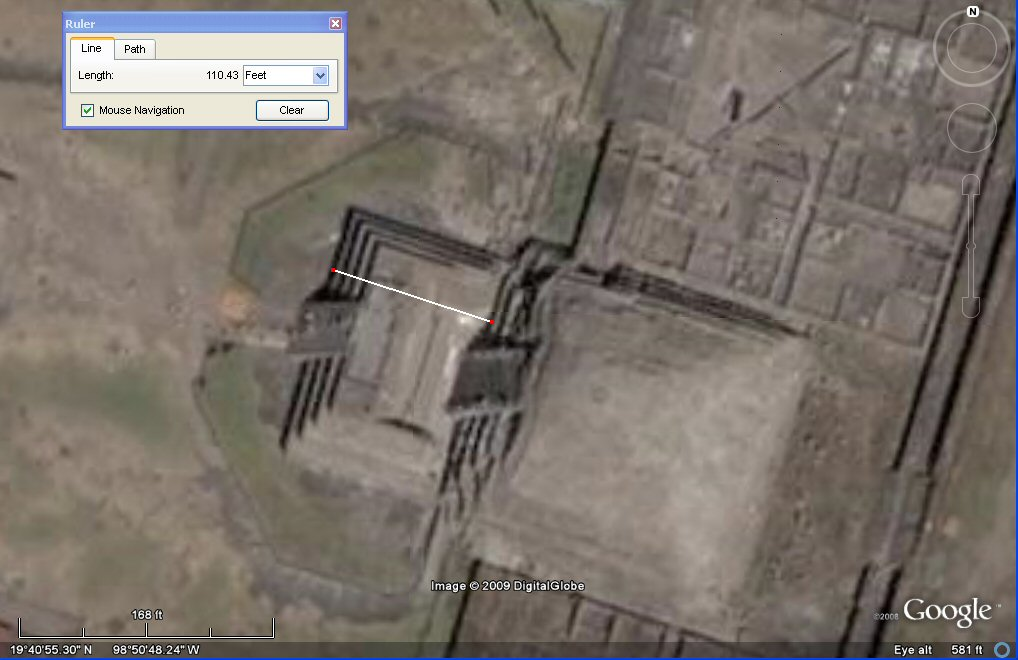above, the Google Earth satellite measures the distance from the front of the front pyramid platform base to the front of the rear pyramid base as 110 English feet = 100 Sumerian feet.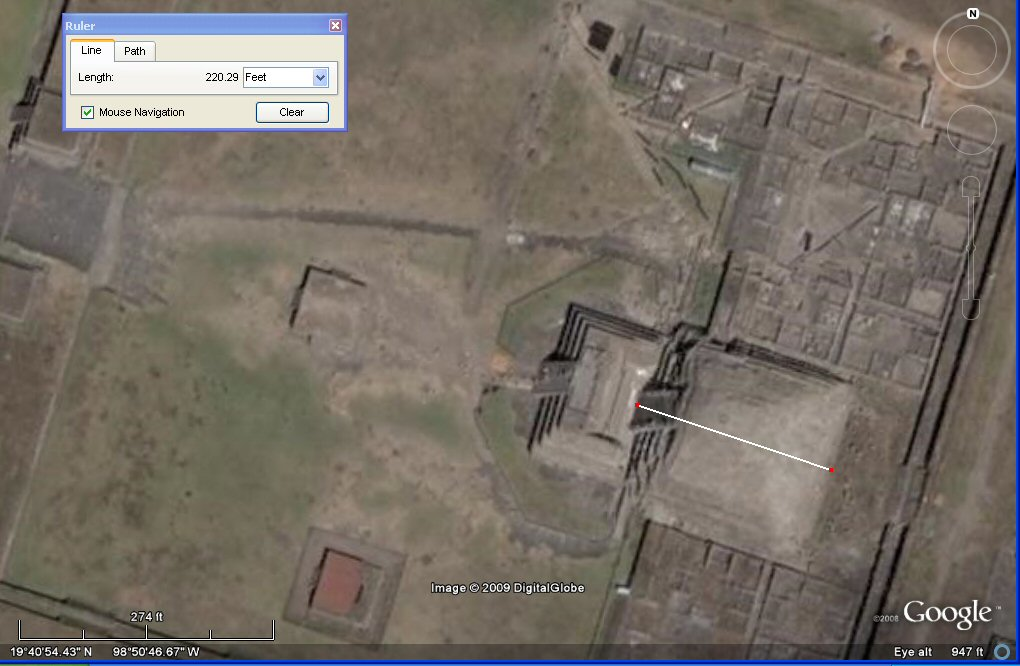above, the Google Earth satellite measures the depth of the pyramid complex as 220 English feet
= 200 Sumerian feet of 13.2"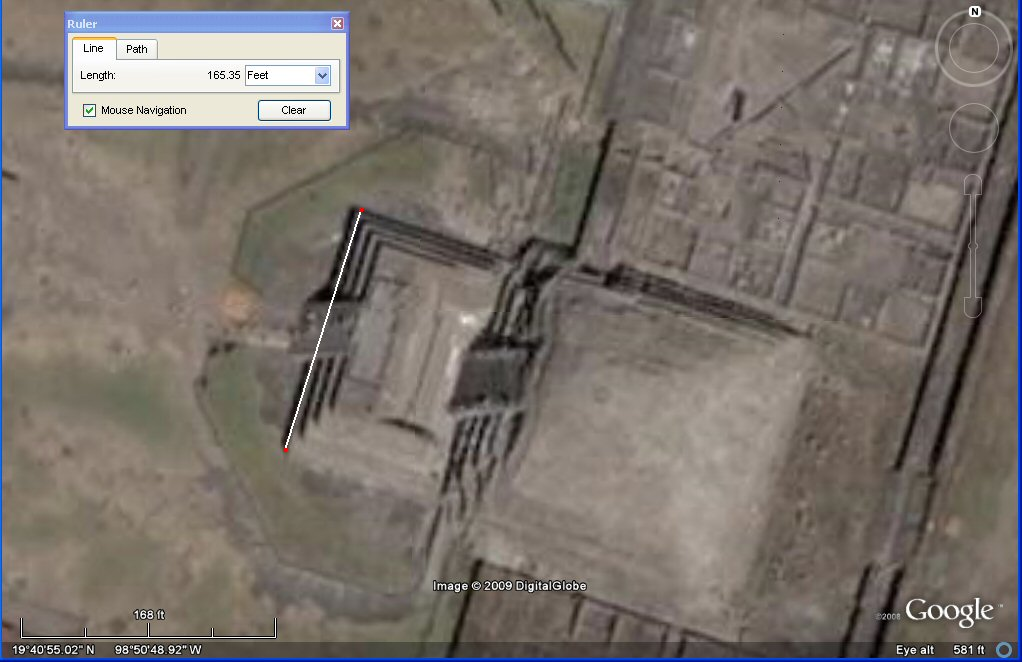above, the Google Earth satellite measures the width of the front pyramid platform base as 165 English feet
= 100 Sumerian cubits of 19.8" which is also 150 Sumerian feet of 19.8", 60 Sumerian yards of 33.0",
96 Egyptian royal cubits of 20.62" or 48 Mayan hunabs of 41.25".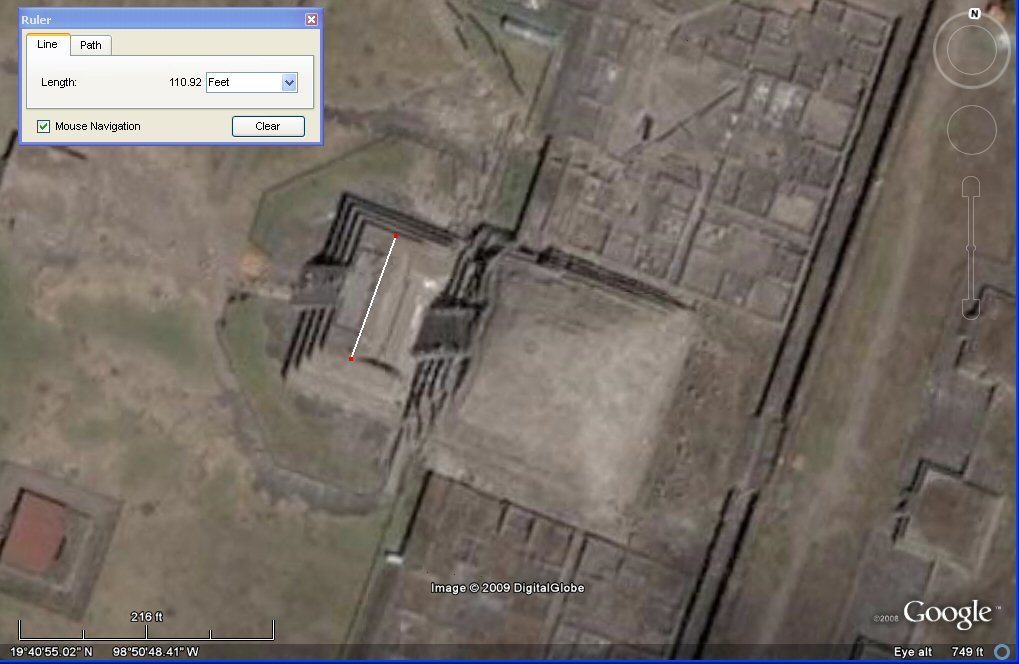above, the Google Earth satellite measures the width of the pyramid platform as 110 English feet = 100 Sumerian feet. .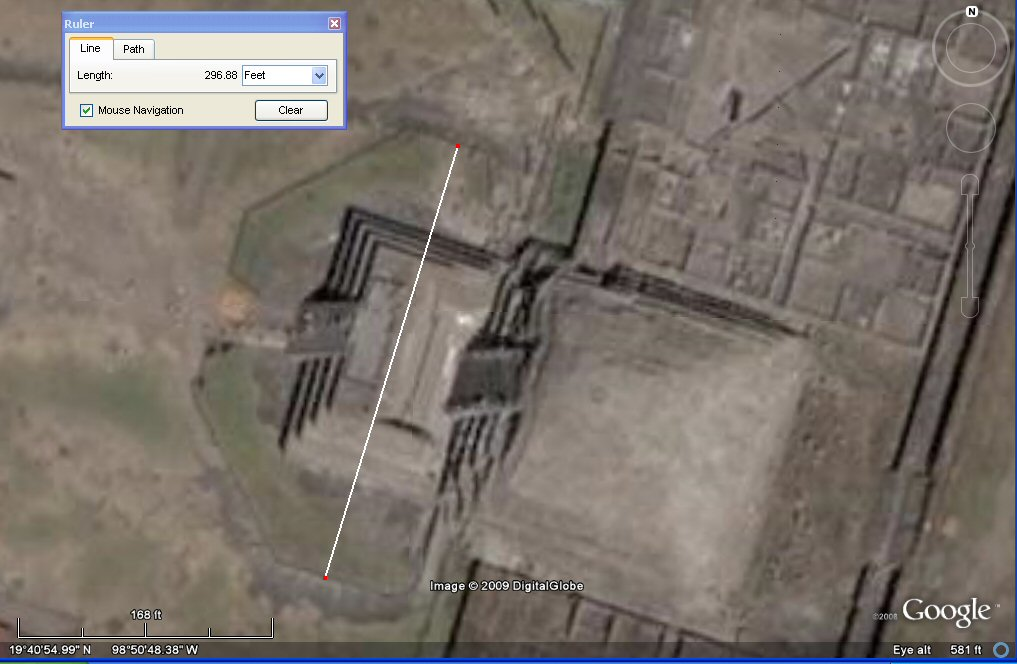above, the Google Earth satellite measures the width of this base as 297 English feet = 180 Sumerian cubits of 19.8"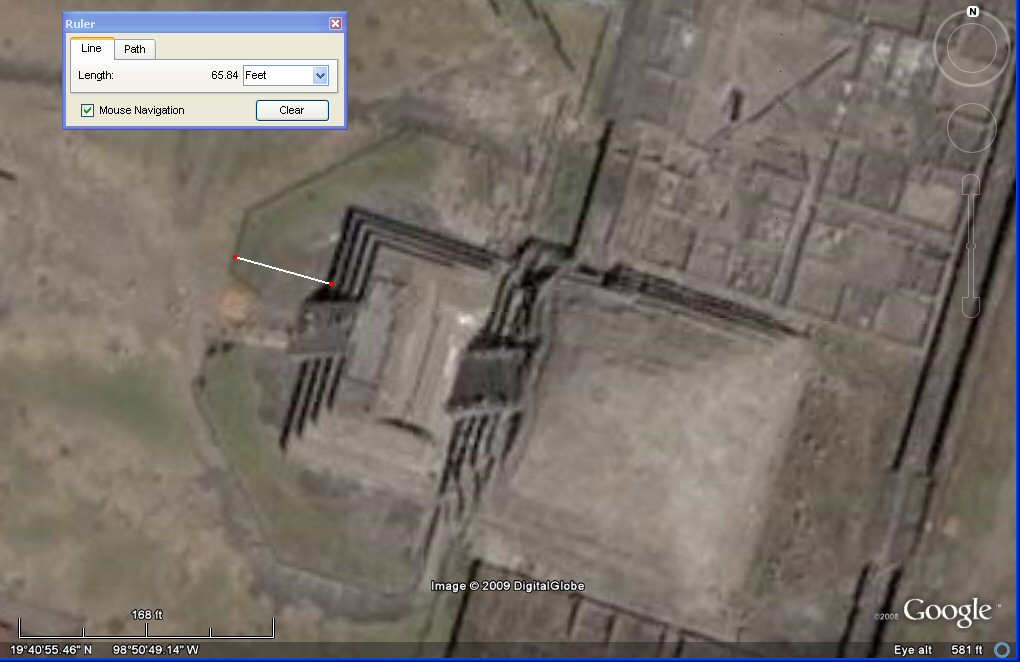above, the Google Earth satellite measures this distance to the front
as 66 English feet = 60 Sumerian feet or 40 Sumerian cubits.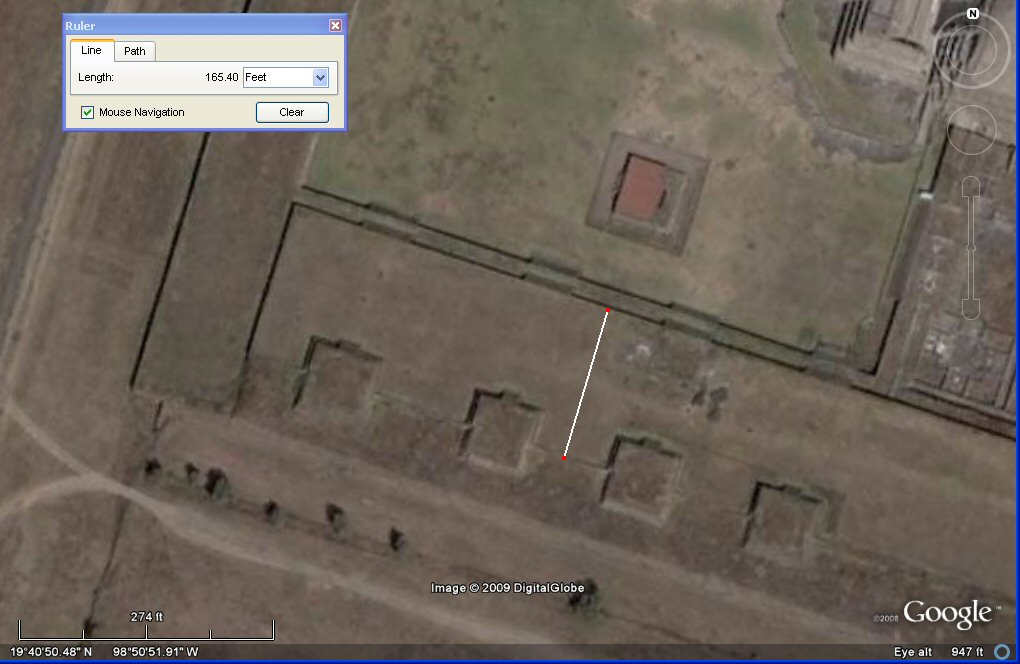above, the Google Earth satellite measures the width of this terrace as 165 English feet
= 100 Sumerian cubits of 19.8".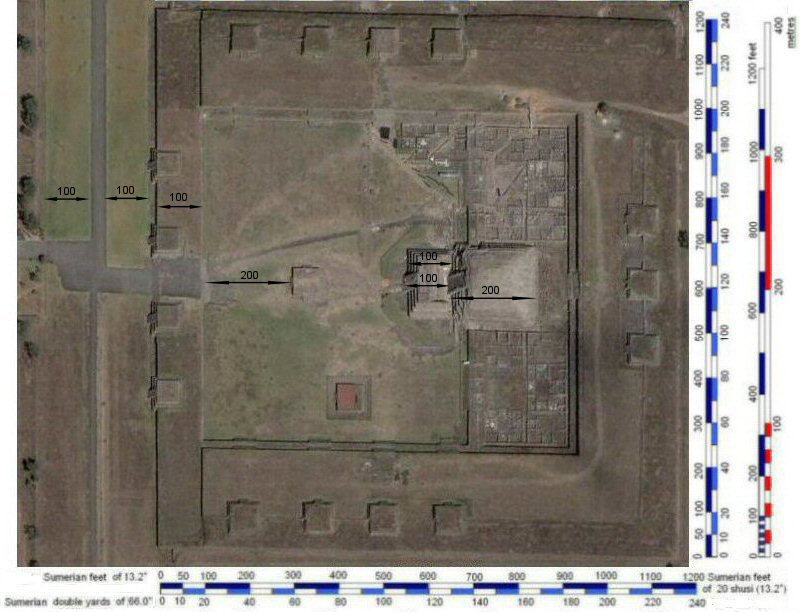above, the Google Earth photo with units in Sumerian feet imposed.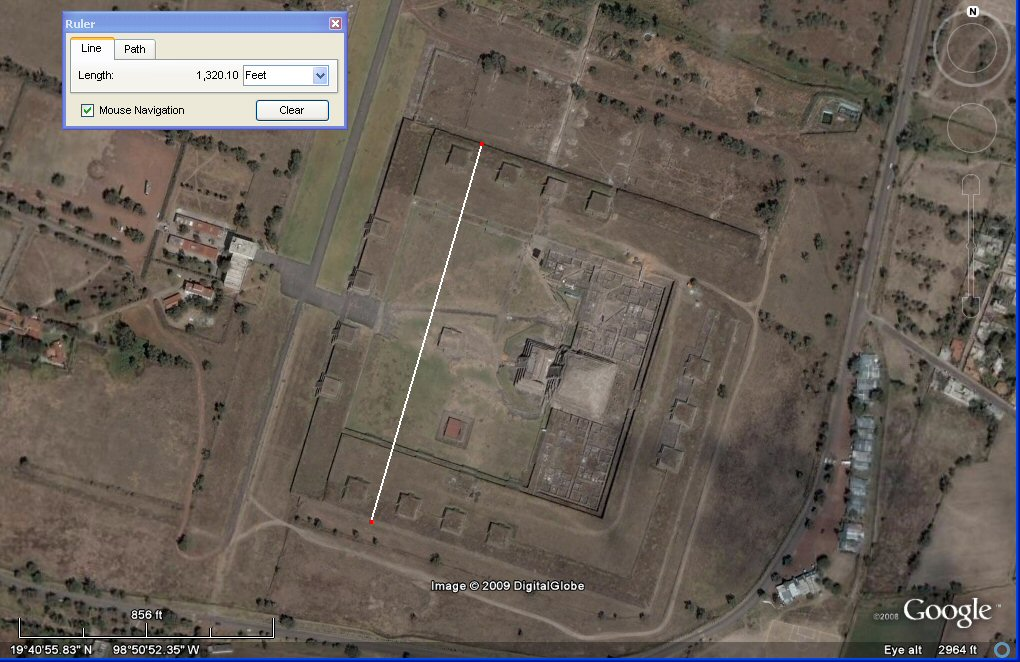above, the Google Earth satellite measures the width of the complex as 1320 english feet
which is 1200 Sumerian feet of 13.2" or 800 Sumerian cubits of 19.8"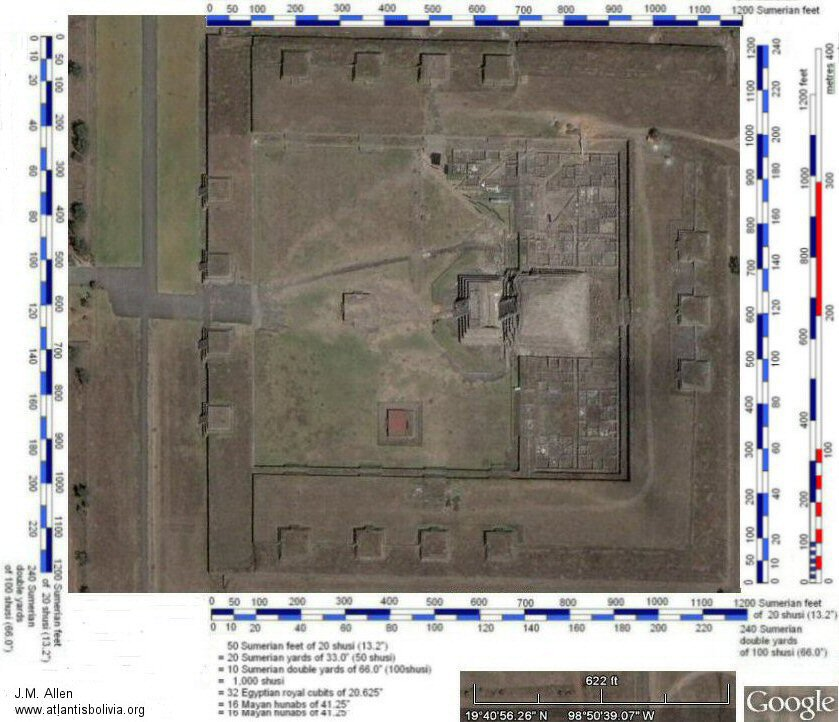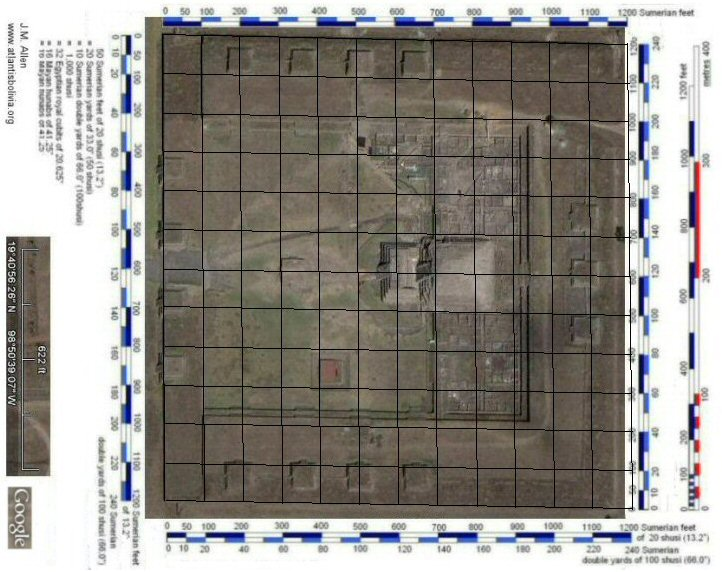above, the Google Earth photo with scaled grid at 100 Sumerian feet intervals.above, the Google Earth photo with scaled grid at 50 Sumerian feet intervals.

From the satellite images, the complex appears to measure 1200 Sumerian feet by 1150 Sumerian feet. Although there are many instances of multiples of 100 Sumerian feet suggestng a grid of 100 Sumerian feet was used in the planning, the odd shape of 1200 x 1150 Sumerian feet suggests it was planned on a grid of 50 Sumerian feet.

A unit of 50 Sumerian feet of 13.2" would be 20 Sumerian yards of 50 shusi, or 10 double yards of 100 shusi, or simply, 1,000 Sumerian shusi of 0.66". It would also be 32 Egyptian royal cubits of 20.625" or 16 Mayan hunabs of 41.25". Surprisingly it also means that 32 Egyptian royal cubits were equal to 1,000 Sumerian shusi, seeming to confirm that all the above measurements although attributed to varying countries as far apart as Sumeria, Egypt and Mexico, were originally part of a unified system as seen at Teotihuacan.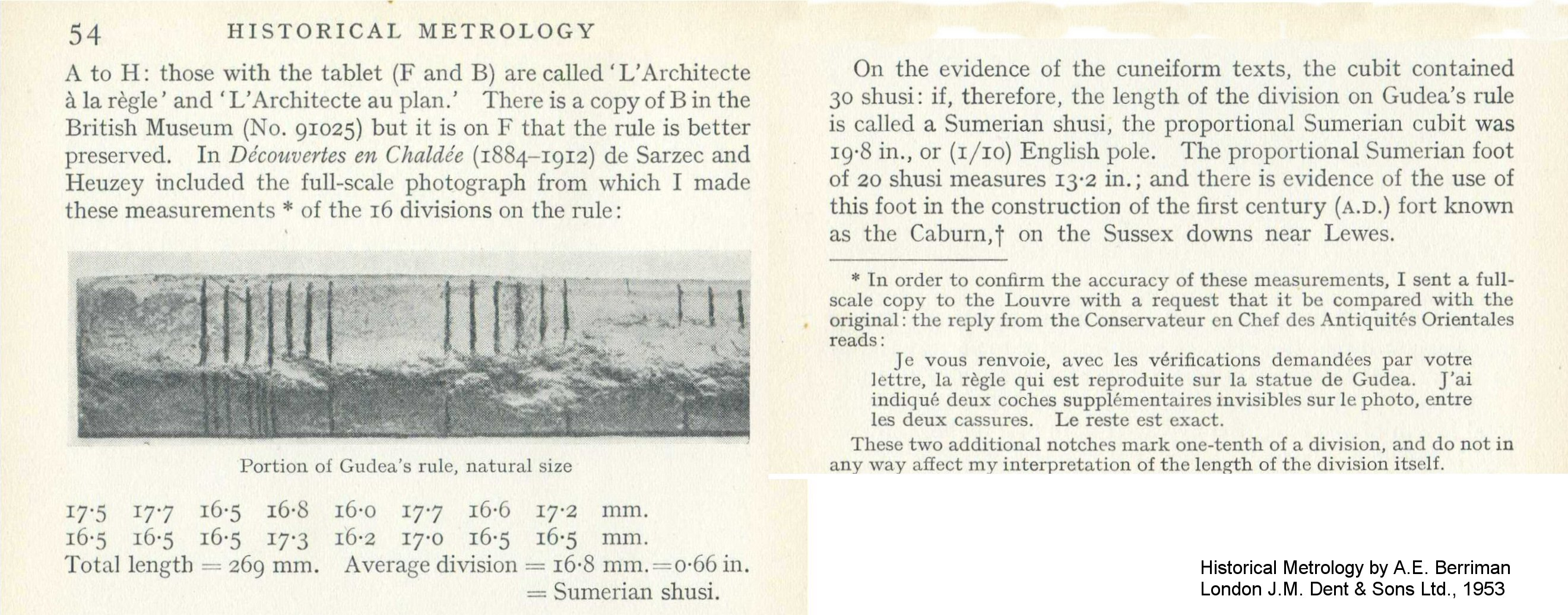above, a portion of the ruler known as "Gudea's rule" preserved in the Louvre shows intervals in Sumerian "shusi" of 0.66" which Berriman demonstrated formed 20 to the Sumerian foot of 13.2" and 30 to the Sumerian cubit of 19.8".
50 shusi made the Sumerian yard of 33.0" and 100 shusi made the Sumerian "double yard".

References
Berriman A.E. (1953) Historical metrology, J.M. Dent & Sons, London 1953.

Chichen Itza and El Castillo cubits

Monte Alban cubits measuring units

Tiwanaku calendar